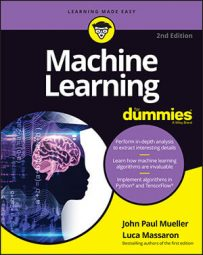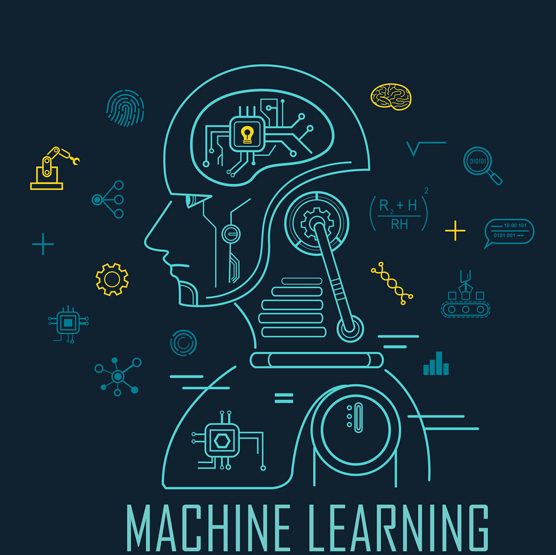##### Machine Learning For DummiesMachine learning is an incredible technology that you use more often than you think today and that has the potential to do even more tomorrow. The interesting thing about machine learning is that Python makes the task easier than most people realize because it comes with a lot of built-in and extended support (through the use of libraries, datasets, and other resources). With that in mind, this Cheat Sheet helps you access the most commonly needed reminders for making your machine learning experience fast and easy.© buffaloboy / Shutterstock.com

## Locate the Algorithm You Need

Machine learning requires the use of a large number of algorithms to perform various tasks. However, finding the specific algorithm you want to know about could be difficult. The following table provides you with an online location for information about the most common algorithms.

 Algorithm Type Python/R URL Naïve Bayes Supervised classification, online learning https://scikit-learn.org/stable/modules/naive_bayes.html PCA Unsupervised https://scikit-learn.org/stable/modules/generated/sklearn.decomposition.PCA.html SVD Unsupervised https://scikit-learn.org/stable/modules/generated/sklearn.decomposition.TruncatedSVD.html K-means Unsupervised https://scikit-learn.org/stable/modules/generated/sklearn.cluster.KMeans.html K-Nearest Neighbors Supervised regression and classification https://scikit-learn.org/stable/modules/neighbors.html Linear Regression Supervised regression, online learning https://scikit-learn.org/stable/modules/generated/sklearn.linear_model.LinearRegression.html Logistic Regression Supervised classification, online learning https://scikit-learn.org/stable/modules/generated/sklearn.linear_model.LogisticRegression.html Neural Networks Unsupervised Supervised regression and classification https://scikit-learn.org/dev/modules/neural_networks_supervised.html Support Vector Machines Supervised regression and classification https://scikit-learn.org/stable/modules/svm.html Adaboost Supervised classification https://scikit-learn.org/stable/modules/generated/sklearn.ensemble.AdaBoostClassifier.html Gradient Boosting Supervised regression and classification https://scikit-learn.org/stable/modules/generated/sklearn.ensemble.GradientBoostingClassifier.html Random Forest Supervised regression and classification https://scikit-learn.org/stable/modules/generated/sklearn.ensemble.RandomForestClassifier.html

## Choose the Right Algorithm

Machine Learning For Dummies, 2nd Edition discusses a lot of different algorithms, and it may seem at times as if it will never run out. The following table provides you with a quick summary of the strengths and weaknesses of the various algorithms.

 Algorithm Best at Pros Cons Random Forest Apt at almost any machine learning problem Bioinformatics Can work in parallel Seldom overfits Automatically handles missing values if you impute using a special number No need to transform any variable No need to tweak parameters Difficult to interpret Weaker on regression when estimating values at the extremities of the distribution of response values Biased in multiclass problems toward more frequent classes Gradient Boosting Apt at almost any machine learning problem Search engines (solving the problem of learning to rank) It can approximate most nonlinear function Best in class predictor Automatically handles missing values No need to transform any variable It can overfit if run for too many iterations Sensitive to noisy data and outliers Doesn’t work at its best without parameter tuning Linear regression * Baseline predictions * Econometric predictions * Modelling marketing responses * Simple to understand and explain * It seldom overfits * Using L1 & L2 regularization is effective in feature selection * Fast to train * Easy to train on big data thanks to its stochastic version * You have to work hard to make it fit nonlinear functions * Can suffer from outliers Support Vector Machines Character recognition Image recognition Text classification Automatic non-linear feature creation Can approximate complex non-linear functions Works only with a portion of the examples (the support vectors) Difficult to interpret when applying  non-linear kernels Suffers from too many examples, after 10,000 examples it starts taking too long to train K-Nearest Neighbors Computer vision Multilabel tagging Recommender systems Spell checking problems Fast, lazy training Can naturally handle extreme multiclass problems (like tagging text) Slow and cumbersome in the predicting phase Can fail to predict correctly due to the curse of dimensionality Adaboost Face detection Automatically handles missing values No need to transform any variable It doesn’t overfit easily Few parameters to tweak It can leverage many different weak-learners Sensitive to noisy data and outliers Never the best in class predictions Naive Bayes Face recognition Sentiment analysis Spam detection Text classification Easy and fast to implement, doesn’t require too much memory and can be used for online learning Easy to understand Takes into account prior knowledge Strong and unrealistic feature independence assumptions Fails estimating rare occurrences Suffers from irrelevant features Neural Networks Image recognition Language recognition and translation Speech recognition Vision recognition It can approximate any non-linear function Robust to outliers It can work with image, text and sound data It requires you to define a network architecture Difficult to tune because of too many parameters and you have also to decide the architecture of the network Difficult to interpret Easy to overfit Logistic regression Ordering results by probability Modelling marketing responses Simple to understand and explain It seldom overfits Using L1 & L2 regularization is effective in feature selection The best algorithm for predicting probabilities of an event Fast to train Easy to train on big data thanks to its stochastic version You have to work hard to make it fit non-linear functions Can suffer from outliers SVD Recommender systems Can restructure data in a meaningful way Difficult to understand why data has been restructured in a certain way PCA Removing collinearity Reducing dimensions of the dataset Can reduce data dimensionality Implies strong linear assumptions (components are a weighted summations of features) K-means Segmentation Fast in finding clusters Can detect outliers in multiple dimensions Suffers from multicollinearity Clusters are spherical, can’t detect groups of other shape Unstable solutions, depends on initialization

## Get the Right Package

When working with Python, you gain the benefit of not having to reinvent the wheel when it comes to algorithms. There is a package available to meet your specific needs—you just need to know which one to use. The following table provides you with a listing of common Python packages. When you want to perform any algorithm-related task, simply load the package needed for that task into your programming environment.

• K-means: cluster.KMeans and sklearn.cluster.MiniBatchKMeans
• K-Nearest Neighbors: neighbors.KNeighborsClassifier and sklearn.neighbors.KNeighborsRegressor
• Linear regression: linear_model.LinearRegression, sklearn.linear_model.Ridge, sklearn.linear_model.Lasso, sklearn.linear_model.ElasticNet, and sklearn.linear_model.SGDRegressor
• Logistic regression: linear_model.LogisticRegression and sklearn.linear_model.SGDClassifier
• Naive Bayes: naive_bayes.GaussianNB. sklearn.naive_bayes.MultinomialNB, and sklearn.naive_bayes.BernoulliNB
• Neural Networks: keras
• Principal Component Analysis (PCA): sklearn.decomposition.PCA
• Random Forest: ensemble.RandomForestClassifier. sklearn.ensemble.RandomForestRegressor, sklearn.ensemble.ExtraTreesClassifier, and sklearn.ensemble.ExtraTreesRegressor
• Support Vector Machines (SVMs): svm.SVC, sklearn.svm.LinearSVC, sklearn.svm.NuSVC, sklearn.svm.SVR, sklearn.svm.LinearSVR, sklearn.svm.NuSVR, and sklearn.svm.OneClassSVM
• Singular Value Decomposition (SVD): decomposition.TruncatedSVD and sklearn.decomposition.NMF

## Differentiating Learning Types

Algorithms are said to learn, but it’s important to know how they learn because they most definitely don’t learn in the same way that humans do. Learning comes in many different flavors, depending on the algorithm and its objectives. You can divide machine learning algorithms into three main groups based on their purpose:

• Supervised learning: Occurs when an algorithm learns from example data and associated target responses that can consist of numeric values or string labels — such as classes or tags — in order to later predict the correct response when posed with new examples. The supervised approach is, indeed, similar to human learning under the supervision of a teacher. The teacher provides good examples for the student to memorize, and the student then derives general rules from these specific examples.
• Unsupervised learning: Occurs when an algorithm learns from plain examples without any associated response, leaving the algorithm to determine the data patterns on its own. This type of algorithm tends to restructure the data into something else, such as new data features that may represent a class or some new values helpful for additional analysis or for the training a predictive model.
• Reinforcement learning: Occurs when you sequentially present the algorithm with examples that lack labels, as in unsupervised learning. However, you accompany each example with positive or negative feedback according to the solution the algorithm proposes. Reinforcement learning is connected to applications for which the algorithm must make decisions (so that the product is prescriptive, not just descriptive, as in unsupervised learning), and the decisions bear consequences.